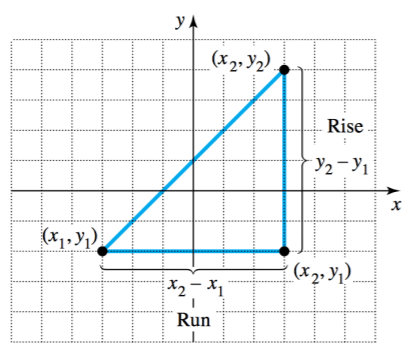# Slope Calculator

Enter the coordinates of two points in the given input boxes. Click the Calculate button to find the slope for given points using slope of a line calculator.## Slope formula

The formula of slope can be expressed in the form of following equation.

m = (y - y) / (x - x)

Where,

m represents the slope,

y - yis change in y (ΔX),

x - x is change in x (ΔY).

To find the equation of line, use our slope intercept form calculator.

Slope calculator is an online tool that calculates the slope of given points in the Cartesian coordinate system. It also finds the angle formed by slope as well as ΔX and ΔY.

## What is a slope of a line?

The slope of a line is a measure of its steepness. In mathematics, slope is calculated as rise over run. In simple words, it is calculated as the change in y divided by change in x.## How to calculate slope?

Apart from using slope finder to calculate slope, you can also find slope of line by using equation of slope.

Example:

For two points (2, 3) and (1, 4), find the slope of line.

Step 1: Write down all values and identify them.

x1 = 2, x2 = 1, y1 = 3, y2 = 4

Step 2: Place the values in slope equation.

m = (y - y) / (x - x)

m = (4 - 3) / (1 - 2)

m = 1/-1

m = -1

1. How use the slope formula and find the slope of a line by mathwarehouse.com